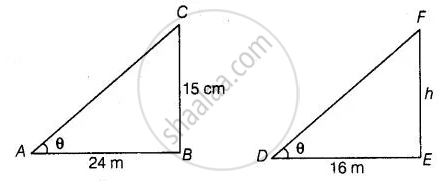# A 15 metres high tower casts a shadow 24 metres long at a certain time and at the same time, a telephone pole casts a shadow 16 metres long. Find the height of the telephone pole. - Mathematics

Sum

A 15 metres high tower casts a shadow 24 metres long at a certain time and at the same time, a telephone pole casts a shadow 16 metres long. Find the height of the telephone pole.

#### Solution

Let BC = 15 m be the tower and its shadow AB is 24 m.

At that time ∠CAB = 8, Again, let EF = h be a telephone pole and its shadow DE = 16 m.

At the same time ∠EDF = 8

Here, ΔASC and ΔDEF both are right-angled triangles.In ΔABC and ΔDEF,

∠CAB = ∠EDF = θ

∠B = ∠E   ......[Each 90°]

∴ ΔABC ∼ ΔDEF  ......[By AAA similarity criterion]

Then, (AB)/(DE) = (BC)/(EF)

⇒ 24/16 = 15/h

∴ h = (15 xx 16)/24 = 10

Hence, the height of the telephone pole is 10 m.

Concept: Similarity of Triangles
Is there an error in this question or solution?

#### APPEARS IN

NCERT Mathematics Exemplar Class 10
Chapter 6 Triangles
Exercise 6.3 | Q 14 | Page 69
Share